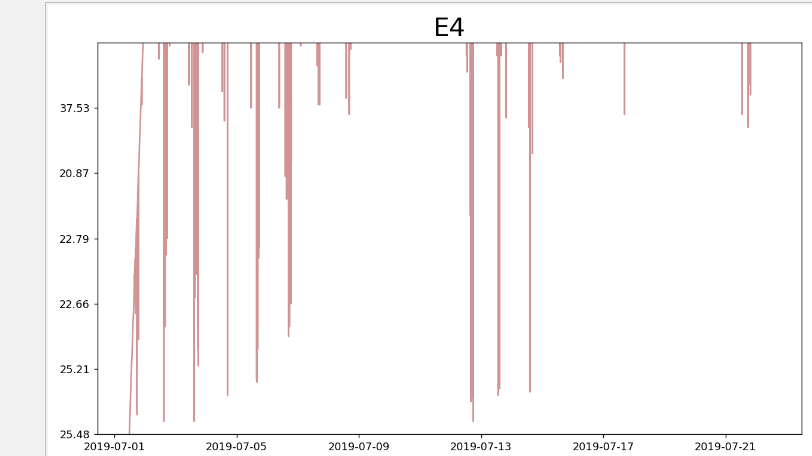matplotlib画图时坐标轴范围和刻度无法正常设置，未报错

import csv
import warnings
warnings.simplefilter(action='ignore', category=FutureWarning)
from datetime import datetime
from matplotlib import pyplot as plt
file_path=r'C:\Users\YSRD-B-0281\Desktop\forth.csv'
with open(file_path) as f:
dates,ohs=[],[]
current_date=datetime.strptime(row,"%Y/%m/%d %H:%M")
dates.append(current_date)
oh=float(row)
ohs.append(row)
fig=plt.figure(dpi=128,figsize=(10,6))
plt.plot(dates,ohs,c='brown',alpha=0.5)
plt.title("E4",fontsize=24)
plt.ylim((0,120))
plt.yticks([0,20,40,60,80,100])
plt.show()
plt.close()MatPlotLib中如何设置坐标轴主刻度线和次刻度线在图的里面呢

python用matplotlib画图时如何使坐标轴的数字显示为千分位的形式如100,000,000

python用matplotlib画图时如何使坐标轴的数字显示为千分位的形式如100,000,000

python画图时，怎么设置坐标轴的单位长度？

python绘图，如何让坐标轴不自动显示加上一个数

python绘图，如何让坐标轴不自动显示加上一个数，如图箭头部分，如何能像图2那样显示。 ![图片说明](https://img-ask.csdn.net/upload/202004/07/1586260694_217635.png) 已解决，步骤如下： ``` import matplotlib.ticker as ticker ax=plt.gca() ax.xaxis.set_major_formatter(ticker.FormatStrFormatter('%.3f')) ax.yaxis.set_major_formatter(ticker.FormatStrFormatter('%.3f')) ```

matplotlib共用x轴标签旋转问题

python 用matplotlib绘图，如何让多个子图的横轴标签全部旋转

matplotlib绘制直方图不显示横坐标值

``` from matplotlib import pyplot as plt import matplotlib matplotlib.rcParams['font.sans-serif']=['SimHei'] # 用黑体显示中文 matplotlib.rcParams['axes.unicode_minus']=False # 正常显示负号 import numpy as np score_array = np.array(score_list) plt.hist(score_array, bins=10) plt.xlabel("区间") plt.ylabel("数量") plt.show() ``` ![图片说明](https://img-ask.csdn.net/upload/201910/15/1571106982_98227.png) 如图可见，直方图横坐标有一条黑线，百思不得其解，求大神解答，万分感谢

python中的画图坐标问题

python使用matplotlib.pyplot绘制折线图纵坐标（y轴）与实际图内坐标不符，请问是什么原因呢？

python 画图，用csv,怎么修改时间间隔和x轴间隔

fig.autofmt_xdate() # 设置x轴时间外观 ax1 = fig.add_subplot(1,1,1) autodate = mdate.AutoDateLocator() ax1.xaxis.set_major_locator(autodate) # 设置时间间隔 # 设置时间标签显示格式 dateFmt = mdate.DateFormatter('%y%m%d') ax1.xaxis.set_major_formatter(dateFmt) # 将x轴次刻度标签设置为61的倍数 xminorLocator = MultipleLocator(61) # 显示次刻度标签的位置,没有标签文本 ax1.xaxis.set_minor_locator(xminorLocator) #ax1.set_xticks() # 设置间隔 ax1.set_xlim(date2num('2018/01/02'),date2num('2018/01/29')) # 设置x轴范围 plt.xticks(rotation=90) # 显示日期旋转90度 plt.title(u'图') plt.plot(x,y, label='') plt.grid(True) plt.xlabel(u'日期') plt.ylabel(u'百分比') plt.legend(loc=2,prop={'family':'SimHei','size':12}) # loc=2 : upper left plt.savefig("图.png", dpi=100) plt.show()

matplotlib,日期，画图，显示不正确

2020年大厂Java面试前复习的正确姿势（800+面试题答案解析）

97年世界黑客编程大赛冠军作品（大小仅为16KB），惊艳世界的编程巨作

A站 AcFun弹幕视频网，简称“A站”，成立于2007年6月，取意于Anime Comic Fun，是中国大陆第一家弹幕视频网站。A站以视频为载体，逐步发展出基于原生内容二次创作的完整生态，拥有高质量互动弹幕，是中国弹幕文化的发源地；拥有大量超粘性的用户群体，产生输出了金坷垃、鬼畜全明星、我的滑板鞋、小苹果等大量网络流行文化，也是中国二次元文化的发源地。 B站 全称“哔哩哔哩(bilibili...

MySQL性能优化(五)：为什么查询速度这么慢